Next: Two-dimensional Laminar Jet. Up: No Title Previous: Approximate Method Based on

# Stagnation Point flow.

For an ideal fluid the flow against an infinite flat plate in the plane y = 0 is given by

 u = Ux, (122)

 v = -Uy, (123)

where U is a constant. When viscosity is included, it still must be true that u is proportional to x, for small x and for all y.Thus, for small x, at least, we may take

 u = kxF(y) (124)

The governing equations for steady flow in terms of dimensional variables are given by equations (2.1) to (2.3). If we substitute equation (7.124) into the continuity equation (2.3), we obtain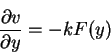(125)

This suggest that we take

 u = kxf'(Ay), (126)

 v = -Bf(Ay). (127)

Now, we substitute equations (7.126) and (7.127) into the governing equations (2.1) to (2.3). We obtain from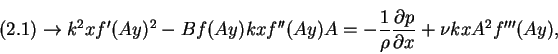(128)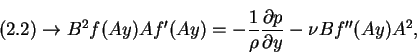(129)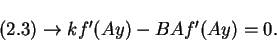(130)

This last equation implies that BA = k, so we can write equations (7.128) and (7.130) in the form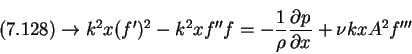(131)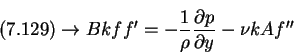(132)

We can solve equation (7.131) for the pressure derivative with respect to x to obtain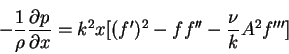(133)

Since the the term between brackets in the right side of the equation above is not a function of x, we set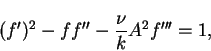(134)

which implies, according to equation (7.133), that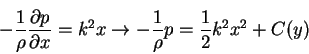(135)

If we substitute equation (7.135) into equation (7.132), we have the relation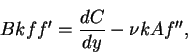(136)

and if we integrate this equation, we obtain that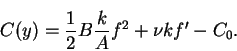(137)

Next, we substitute the expression for C(y) above into the equation (7.135) for the pressure, which gives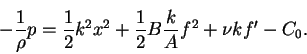(138)

To simplify equation (7.134), we chose the coefficient of f''' equal to one, which implies that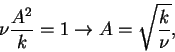(139)

and since BA = k, we have that(140)

With equations (7.138) and (7.139), we can write the equation (7.138) as follows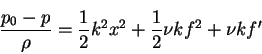(141)

with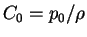and p0 is the pressure as. The function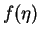, where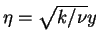satisfies the ordinary differential equation

 (f')2-ff''-f'''-1 = 0 (142)

with boundary conditions:

• No slip condition at the plate surface.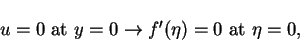(143)

• No flux across the plate: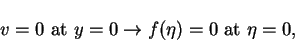(144)

• At, we have u = kx, which implies that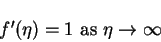(145)

In summary, the functionis the solution of the boundary value problem given by the equations (7.142) to (7.145), which has no closed form solution. The equation ordinary differential equation (7.142) is non-linear and has to be solved numerically together with the boundary conditions (7.143) to (7.145). Figure 2 below illustarte the result of the numerical evaluation of the boundary value problem given by equations (7.142) to (7.145) for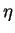in the range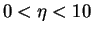.Once we know the values of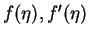and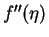, we can obtain the velocities u and v given, respectivelly, by equations (7.126) and (7.127) with B and A given, respectively, by equations (7.139) and (7.140), the pressure field is given by equation (7.141).Next: Two-dimensional Laminar Jet. Up: No Title Previous: Approximate Method Based on
Karl P Burr
2003-03-12